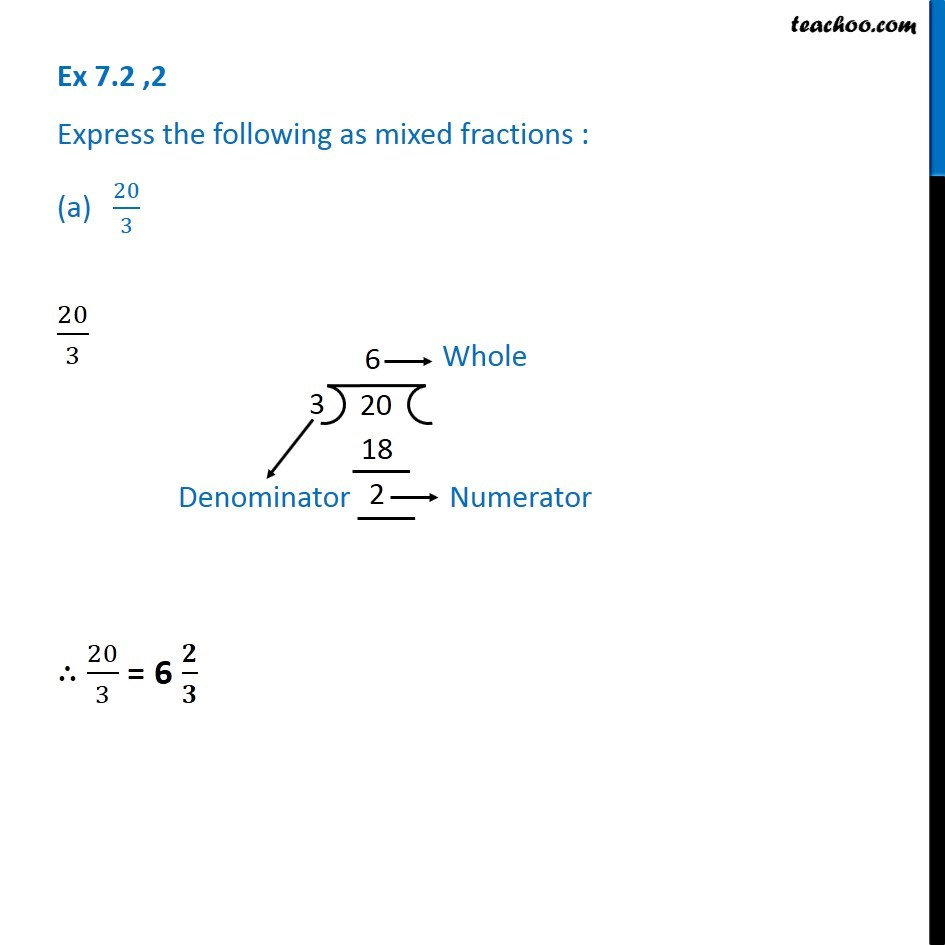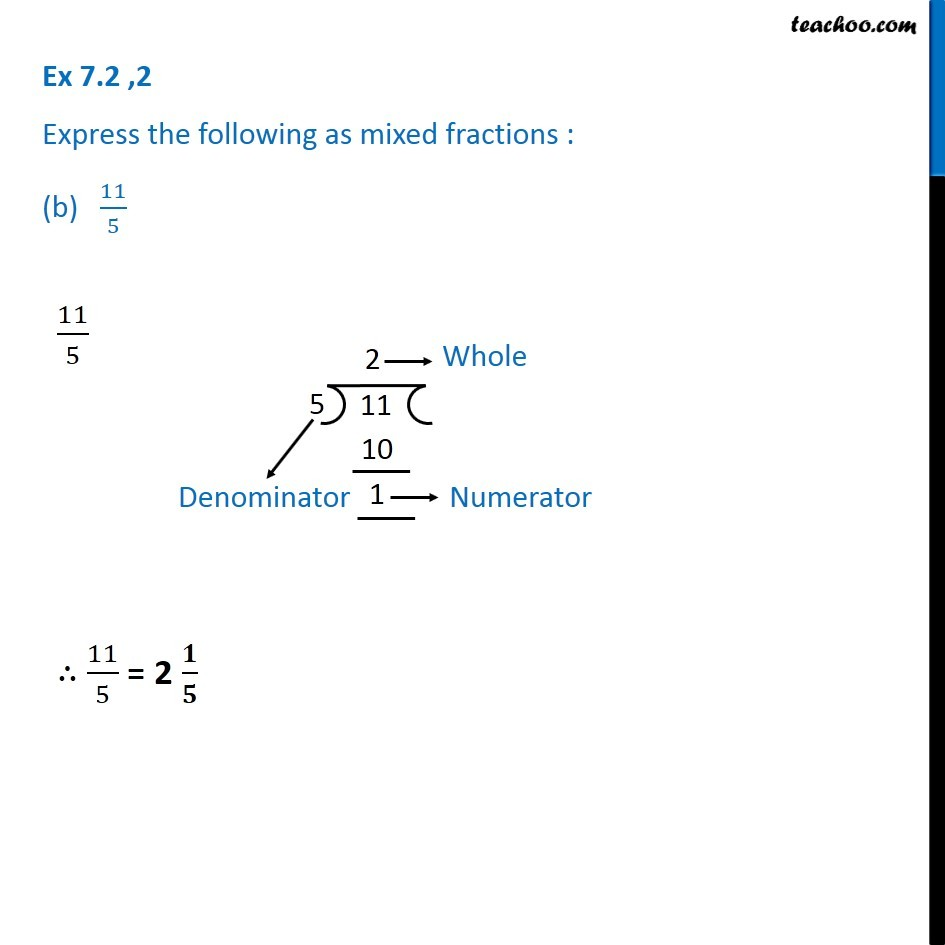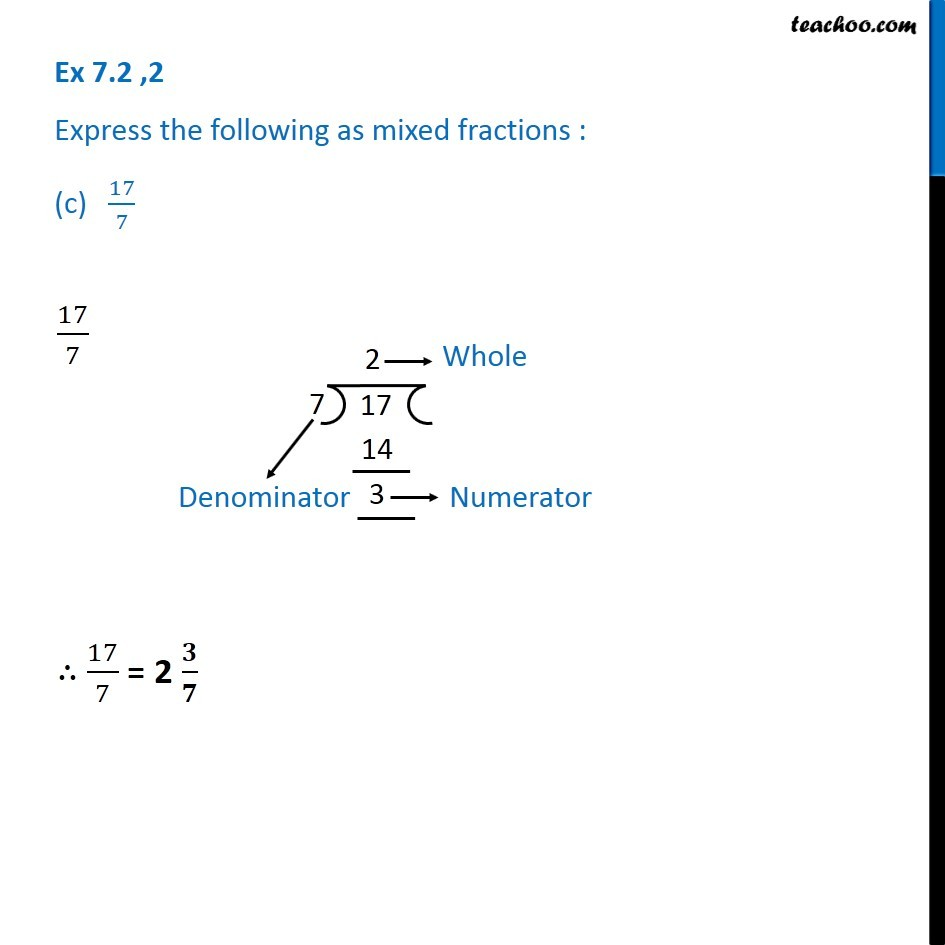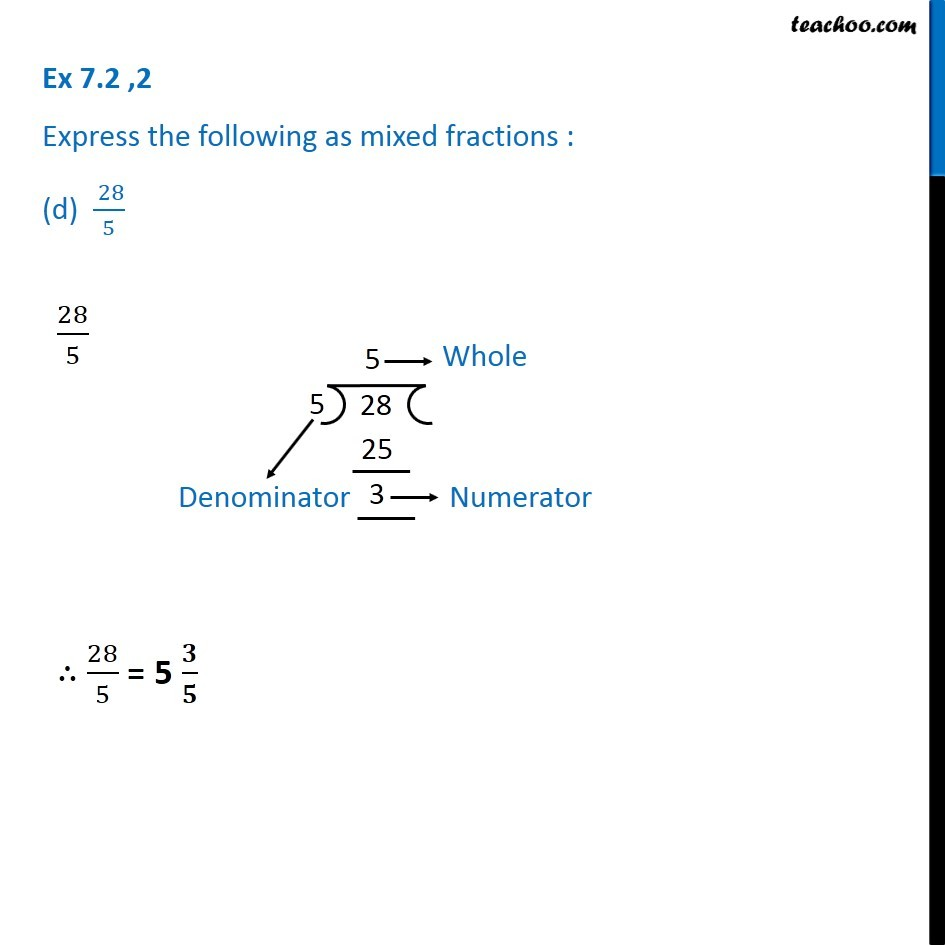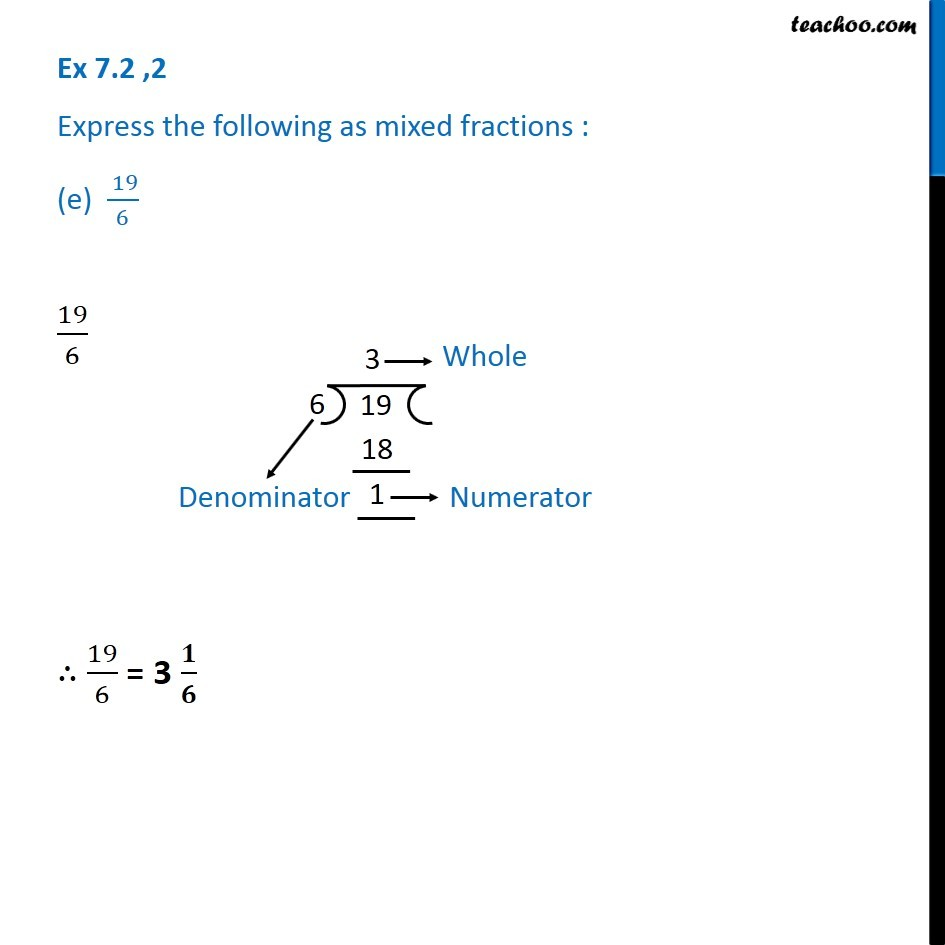Subscribe to our Youtube Channel - https://you.tube/teachoo

1. Chapter 7 Class 6 Fractions
2. Concept wise
3. Proper, improper and mixed fraction

Transcript

Ex 7.2 ,2 Express the following as mixed fractions : (a) 20/3 20/3 ∴ 20/3 = 6 𝟐/𝟑 Ex 7.2 ,2 Express the following as mixed fractions : (b) 11/5 11/5 ∴ 11/5 = 2 𝟏/𝟓 Ex 7.2 ,2 Express the following as mixed fractions : (c) 17/7 17/7 ∴ 17/7 = 2 𝟑/𝟕 Ex 7.2 ,2 Express the following as mixed fractions : (d) ( 28)/5 28/5 ∴ 28/5 = 5 𝟑/𝟓 Ex 7.2 ,2 Express the following as mixed fractions : (e) ( 19)/6 19/6 ∴ 19/6 = 3 𝟏/𝟔 Ex 7.2 ,2 Express the following as mixed fractions : (f) 35/9 35/9 ∴ 35/9 = 3𝟖/𝟗

Proper, improper and mixed fraction